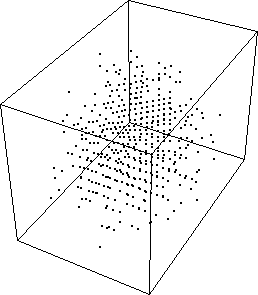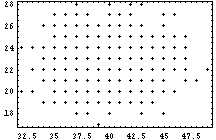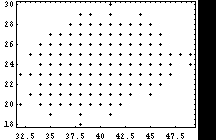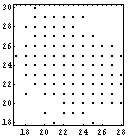# Mathematica and Self-Reference

Some self-documenting sentences (and such) generated with Mathematica:
• `{6,2,1,0,0,0,1,0,0,0}` lists its own count of each digit 0–9.
• `{7,1,0,1,0,0,1,0,0,0}` and `{6,3,0,0,0,0,0,1,0,0}` list each other’s counts of each digit 0–9.
• Four contains four letters.
• This sentence uses nineteen vowels and thirty-four consonants.
• This Mathematica-generated sentence contains sixty-six characters.
• This Mathematica-generated sentence contains sixty-eight characters.
• This Mathematica-generated sentence contains nine e’s.
• This Mathematica-generated sentence contains eleven e’s.
• This Mathematica-generated sentence uses eighteen different letters of the alphabet.
• This Mathematica-generated sentence uses twenty different letters of the alphabet.
• This Mathematica-generated sentence contains seven a’s, one b, four c’s, three d’s, thirty-nine e’s, six f’s, three g’s, ten h’s, thirteen i’s, one j, one k, three l’s, three m’s, twenty-two n’s, twelve o’s, one p, one q, eleven r’s, twenty-eight s’s, twenty-five t’s, four u’s, six v’s, six w’s, four x’s, five y’s, and one z.

While searching for the last sentence, I was hoping there would be some striking correlations among the simultaneous counts of letters—say, t’s, e’s, and n’s—as the iteration went along (something like the Lozi attractor, perhaps), but it doesn’t look like it.

For what it’s worth:

Plot of t vs. e vs. n:Plots of e-vs.-t, e-vs.-n, and t-vs.-n:From one successful search that took 807 iterations:

e t n
Final 39 25 22
Mean 39.9722.5623.76
Variance 8.17 3.59 4.01

See, of course, Hofstadter, D. R., Metamagical Themas; Chapter 2, “Self-Referential Sentences: A Follow-Up”, and Chapter 16, “Mathematical Chaos and Strange Attractors”; Basic Books, New York, 1985.